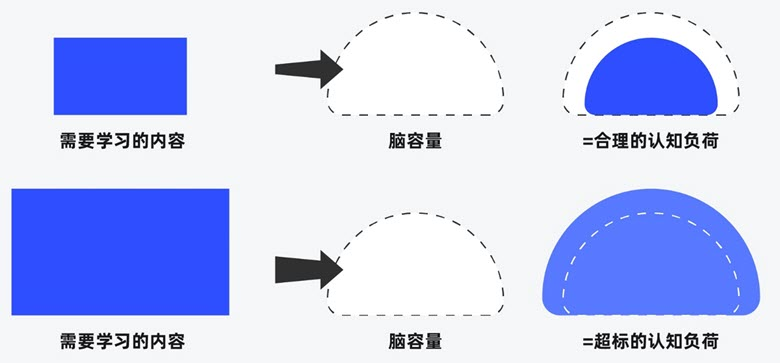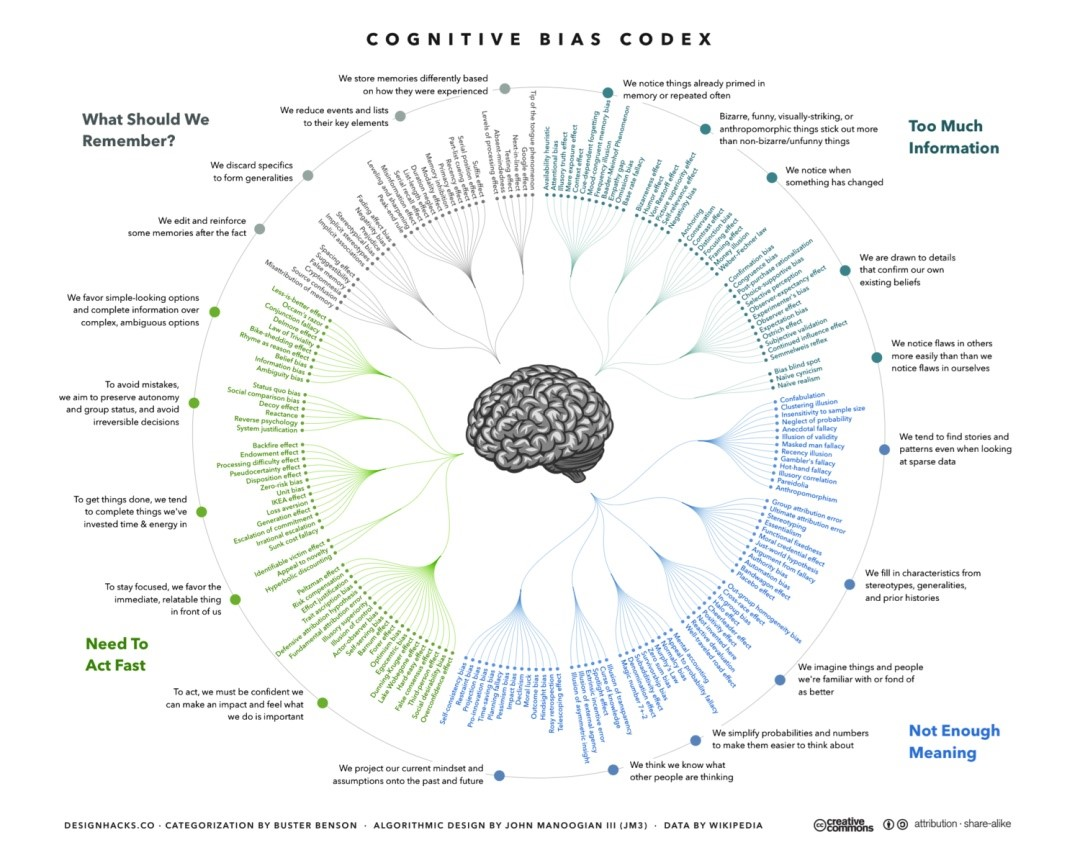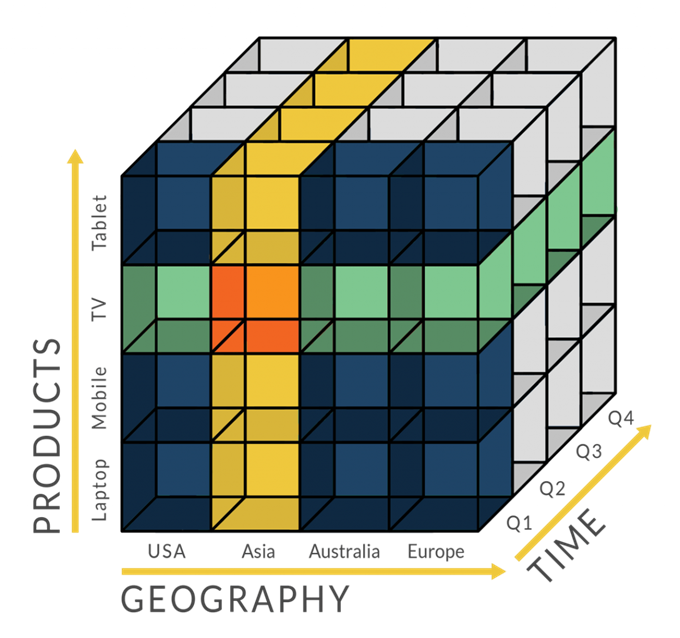# 多层全连接神经网络与情感分析

## 全连接神经网络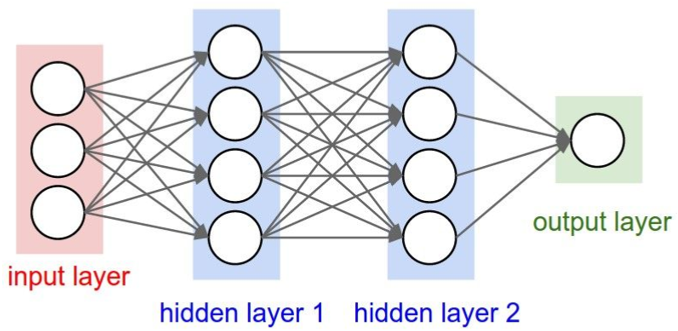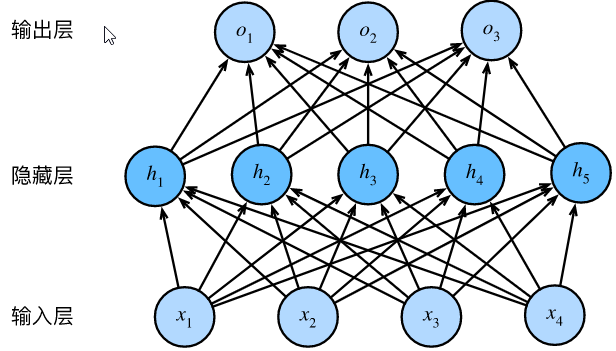$$\boldsymbol{H} = \boldsymbol{X} \boldsymbol{W}_h + \boldsymbol{b}_h,$$

$$\boldsymbol{O} = \boldsymbol{H} \boldsymbol{W}_o + \boldsymbol{b}_o,$$

$$\boldsymbol{O} = (\boldsymbol{X} \boldsymbol{W}_h + \boldsymbol{b}_h)\boldsymbol{W}_o + \boldsymbol{b}_o = \boldsymbol{X} \boldsymbol{W}_h\boldsymbol{W}_o + \boldsymbol{b}_h \boldsymbol{W}_o + \boldsymbol{b}_o.$$

### 激活函数

ReLU函数

ReLU（rectified linear unit）函数提供了一个很简单的非线性变换。给定元素 x ，该函数定义为：$\text{ReLU}(x) = \max(x, 0).$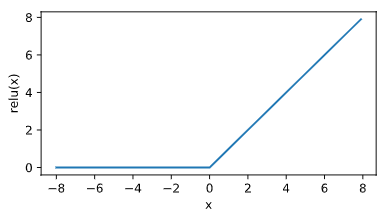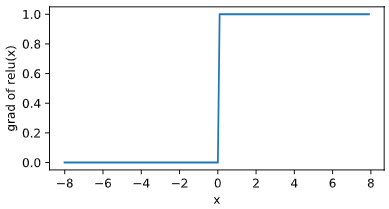sigmoid函数

sigmoid函数可以将元素的值变换到0和1之间：$\text{sigmoid}(x) = \frac{1}{1 + \exp(-x)}.$

sigmoid函数在早期的神经网络中较为普遍，但它目前逐渐被更简单的ReLU函数取代。下面绘制了sigmoid函数。当输入接近0时，sigmoid函数接近线性变换。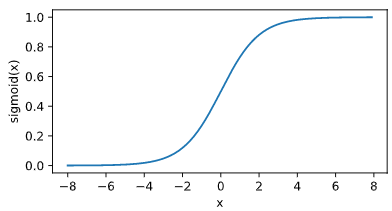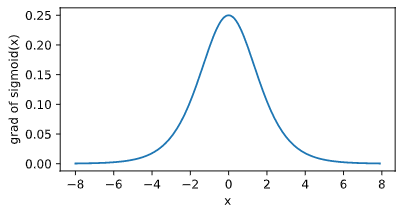tanh函数

tanh（双曲正切）函数可以将元素的值变换到-1和1之间：$\text{tanh}(x) = \frac{1 – \exp(-2x)}{1 + \exp(-2x)}.$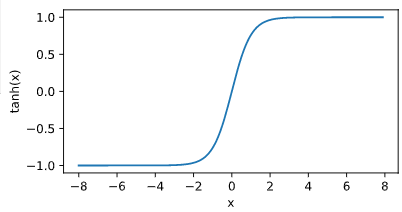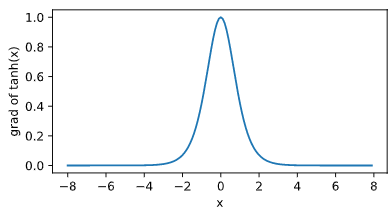### 全连接网络的实现

$$w = \frac{\sum_{i=1}^m y_i(x_i – \overline{x})}{\sum_{i=1}^m x_i^2 – \frac{1}{m} (\sum_{i=1}^m x_i)^2}$$

$$b = \frac{1}{m} \sum_{i=1}^m (y_i – w x_i)^2$$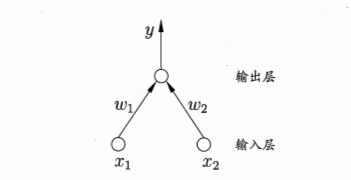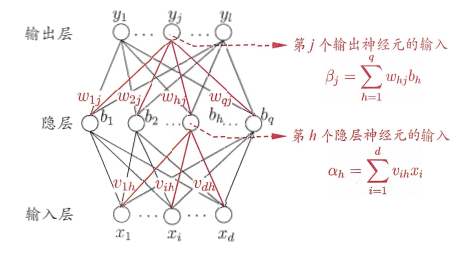$$f’(x) = f(x)(1 – f(x))$$

$$\begin{split} g_j &= -\frac{\partial E_k}{\partial \hat{y}_j^k} \cdot \frac{\partial \hat{y}_j^k}{\partial \beta_j}\ &= -(\hat{y}_j^k – y_j^k)f’(\beta_j – \theta_j) \ &= \hat{y}_j^k (1 – \hat{y}_j^k)(y_j^k – \hat{y}_j^k)\end{split}$$

$$\Delta w_{hj} = \eta g_j b_h$$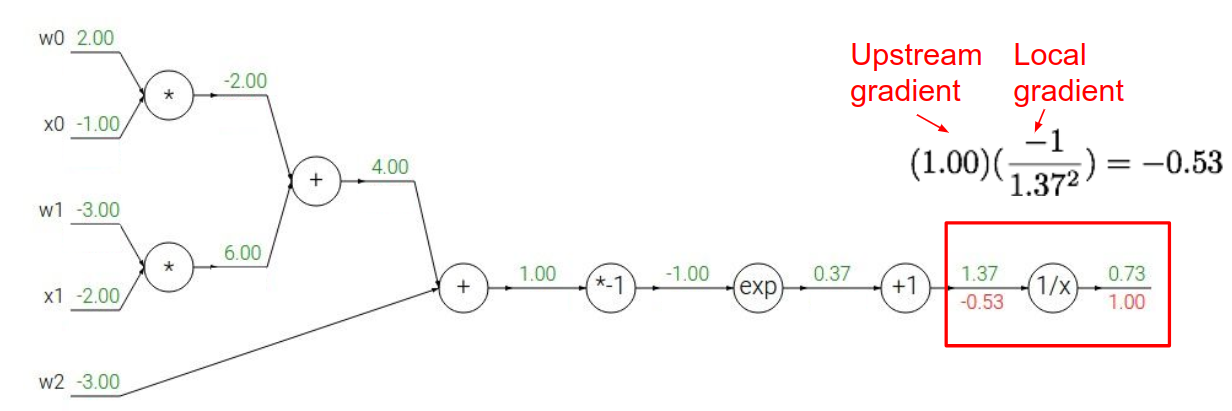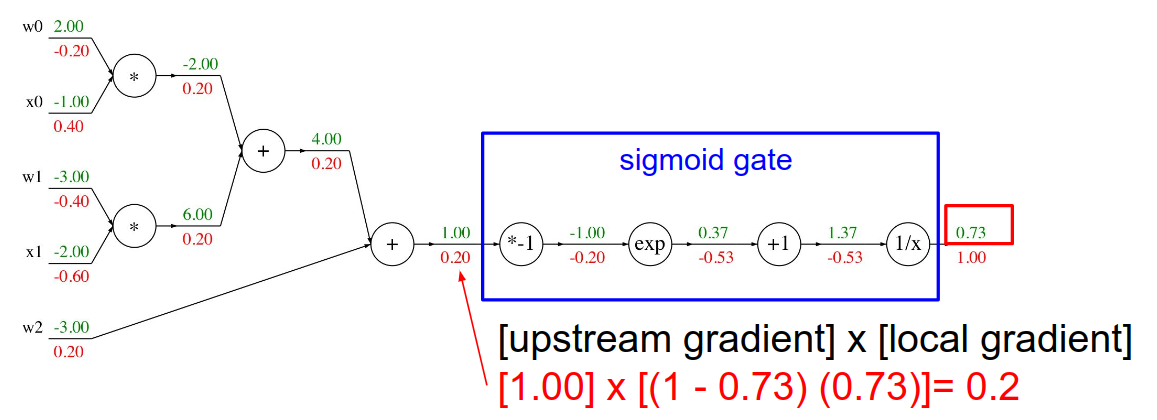## 多层全连接神经网络训练情感分析

### Keras 自带的imdb情感数据

import numpy as np
from keras.datasets import imdb
import matplotlib.pyplot as plt

print(X_train)  # 每个词标注了一个索引(index)，创建了字典。每段文字的每个词对应了一个数字。
print(y_train[:10])  # y 就是标注，1 表示正面，0 表示负面。
print(X_train.shape)
print(y_train.shape)
avg_len = list(map(len, X_train))
print(np.mean(avg_len))
print(max(list(map(len, X_train))))
print(max(list(map(len, X_test))))

plt.hist(avg_len, bins=range(min(avg_len), max(avg_len) + 50, 50))
plt.show()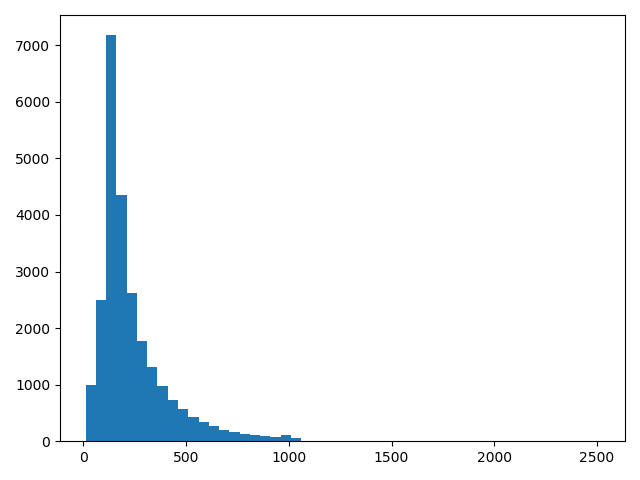Keras 提供了设计嵌入层（Embedding Layer）的模板。只要在建模的时候加一行Embedding Layer 函数的代码就可以。注意，嵌入层一般是需要通过数据学习的，也可以借用已经训练好的嵌入层比如Word2Vec 中预训练好的词向量直接放入模型，或者把预训练好的词向量作为嵌入层初始值，进行再训练。

from keras.models import Sequential
from keras.layers import Dense, Flatten
from keras.layers.embeddings import Embedding
from keras.preprocessing import sequence
import numpy as np
from keras.datasets import imdb

(X_train, y_train), (X_test, y_test) = imdb.load_data()

max_word = 400
vocab_size = np.max([np.max(X_train[i]) for i in range(X_train.shape)]) + 1

model = Sequential()

print(model.summary())

model.fit(X_train, y_train, validation_data=(X_test, y_test), epochs=20, batch_size=100, verbose=1)
score = model.evaluate(X_test, y_test)
print(score)


Model: "sequential_1"
_________________________________________________________________
Layer (type)                 Output Shape              Param #
=================================================================
embedding_1 (Embedding)      (None, 400, 64)           5669568
_________________________________________________________________
flatten_1 (Flatten)          (None, 25600)             0
_________________________________________________________________
dense_1 (Dense)              (None, 2000)              51202000
_________________________________________________________________
dense_2 (Dense)              (None, 500)               1000500
_________________________________________________________________
dense_3 (Dense)              (None, 200)               100200
_________________________________________________________________
dense_4 (Dense)              (None, 50)                10050
_________________________________________________________________
dense_5 (Dense)              (None, 1)                 51
=================================================================
Total params: 57,982,369
Trainable params: 57,982,369
Non-trainable params: 0
_________________________________________________________________


• 第一层是嵌入层，定义了嵌入层的矩阵为vocab_size 64。每个训练段落为其中的max_word 64矩阵，作为数据的输入，填入输入层。
• 然后把输入层压平，原来是max_word * 64 的矩阵，现在变成一维的长度为max_word * 64的向量。
• 接下来不断搭建全连接神经网络，使用relu 函数。
• 最后一层用Sigmoid，预测0，1 变量的概率，类似于logistic regression 的链接函数，目的是把线性变成非线性，并把目标值控制在0~1。因此这里计算的是最后输出的是0 或者1 的概率。